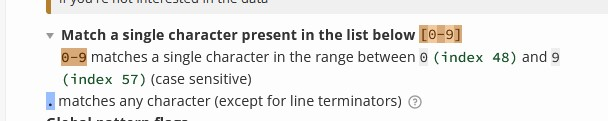# I don't understand why I'm not extracting the correct float

I should be extracting the float 0.875, but I get 0.0

I don’t know why. I’ll post my code below and a link to the challenge. I can’t figure out why I’m not getting the correct answer. Can you help please?

``````import re
handle = open('mbox-short.txt')
numlst = list()
for line in handle:
line = line.rstrip()
stuff = re.findall('^X-DSPAM-Confidence: ([0-9]+)', line)
if len(stuff) != 1: continue
num = float(stuff)
numlst.append(num)
print('Maximum: ', max(numlst))

``````

https://www.freecodecamp.org/learn/scientific-computing-with-python/python-for-everybody/regular-expressions-practical-applications

If I print `numlst` by itself:

‘>>print(numlst)
[0.0, 0.0, 0.0, 0.0, 0.0, 0.0, 0.0, 0.0, 0.0, 0.0, 0.0, 0.0, 0.0, 0.0, 0.0, 0.0, 0.0, 0.0, 0.0, 0.0, 0.0, 0.0, 0.0, 0.0, 0.0, 0.0, 0.0]’

What will your regular expression do if it encounters a decimal? eg. 0.85

So the problem was I didn’t include the decimal. I did and it worked but I have a question/clarification. Please correct me if my assumption is wrong.

A.

`````` stuff = re.findall('^X-DSPAM-Confidence: ([0-9].+)', line)
``````

This checks for any string beginning with ‘X-DSPAM-Confidence:’ that is followed by a colon and any amoung of numbers 0-9 followed by or including a decimal.

B.

`````` stuff = re.findall('^X-DSPAM-Confidence: ([0-9].)+', line)
``````

This checks for any amount of numbers followed by a decimal.
For A. I get ‘.0997’,
B I get ‘78.0’

Is my explanation correct?

Not quite, but I can see the logic there.

``````'^X-DSPAM-Confidence: ([0-9].)+'
``````

`.` matches any character, not a decimal. It’s capturing the last 2 digits of those lines

``````X-DSPAM-Confidence: 0.8475
``````

Just the 75

You can use this great site to test your expressions and it will highlight and explain the results: https://regex101.com/1 Like

[0-9]+

The square bracket is like OR.

if i want to catch also a character ‘B’, i would just throw it in the bracket.
[0-9B]

if i want to catch also characters of ‘A’ or ‘C’. i would do:
[0-9AC]

you can read more at here >Python Regex at W3schools

1 Like